Case Based Questions (MCQ)

Chapter 6 Class 12 Application of Derivatives
Serial order wise

## The Relation between the height of the plant (y in cm) with respect to exposure to sunlight is governed by the following equation y = 4x – 1/2  x 2 where x is the number of days exposed to sunlight.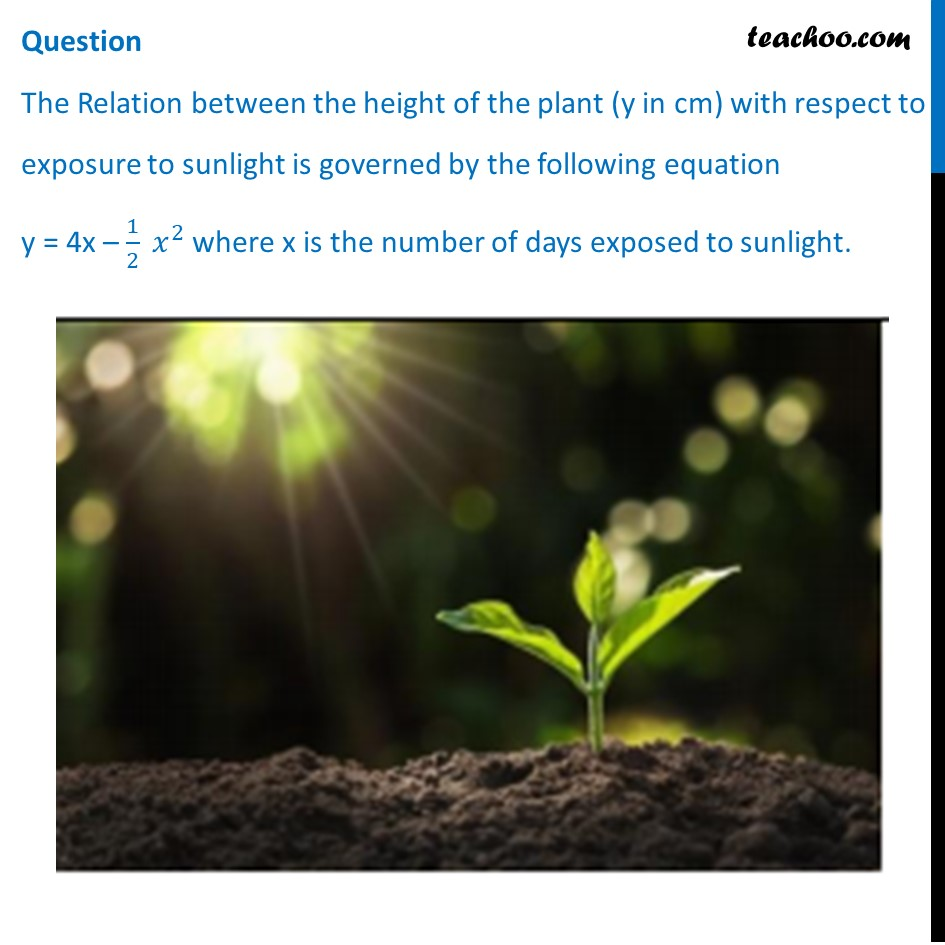## (d) x – 1/2  x 2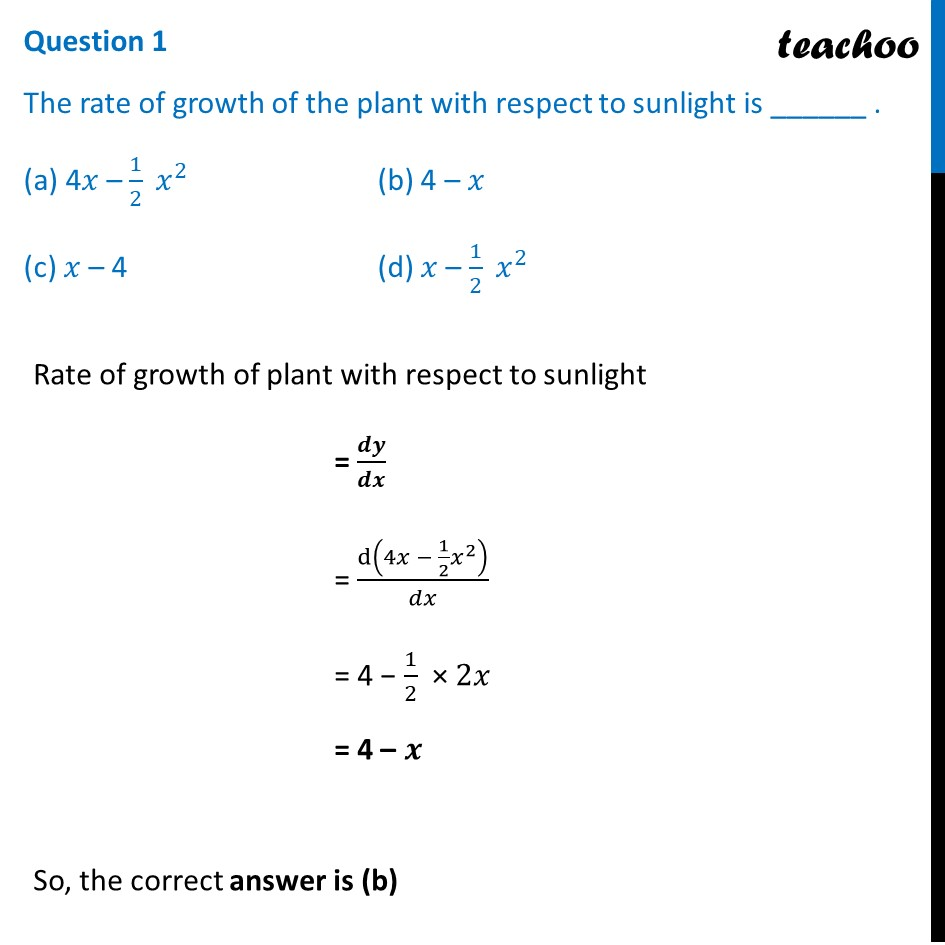## (d) 10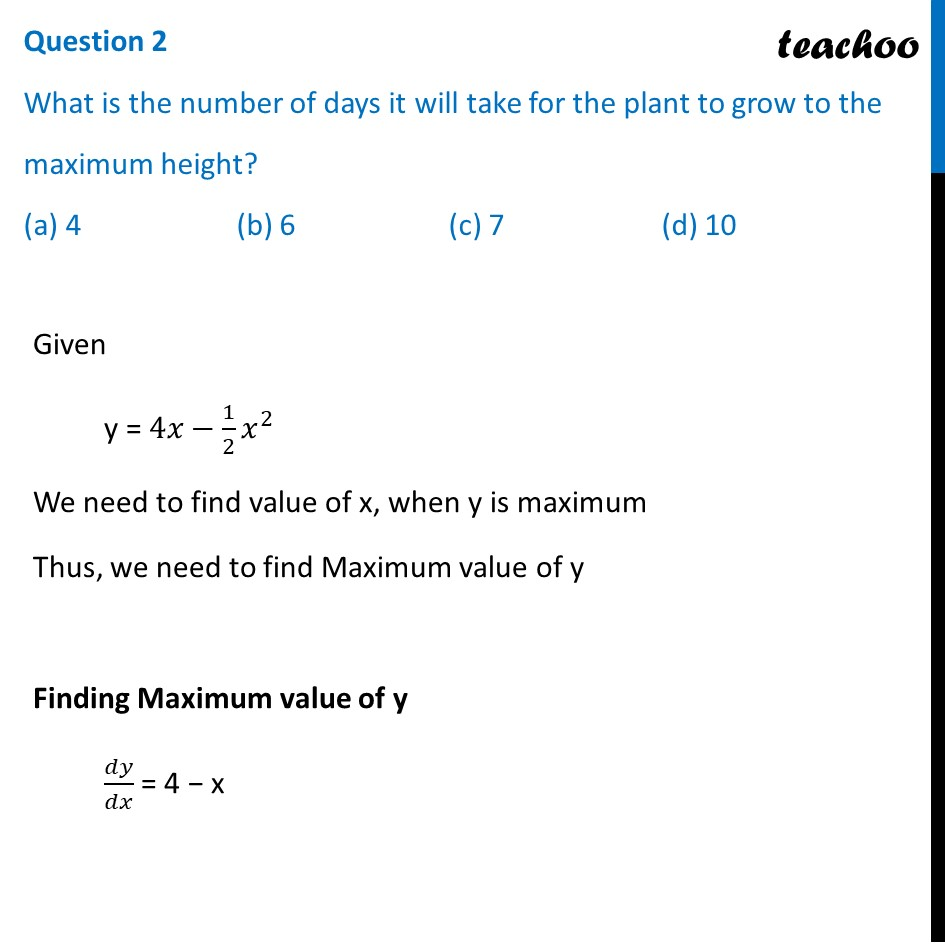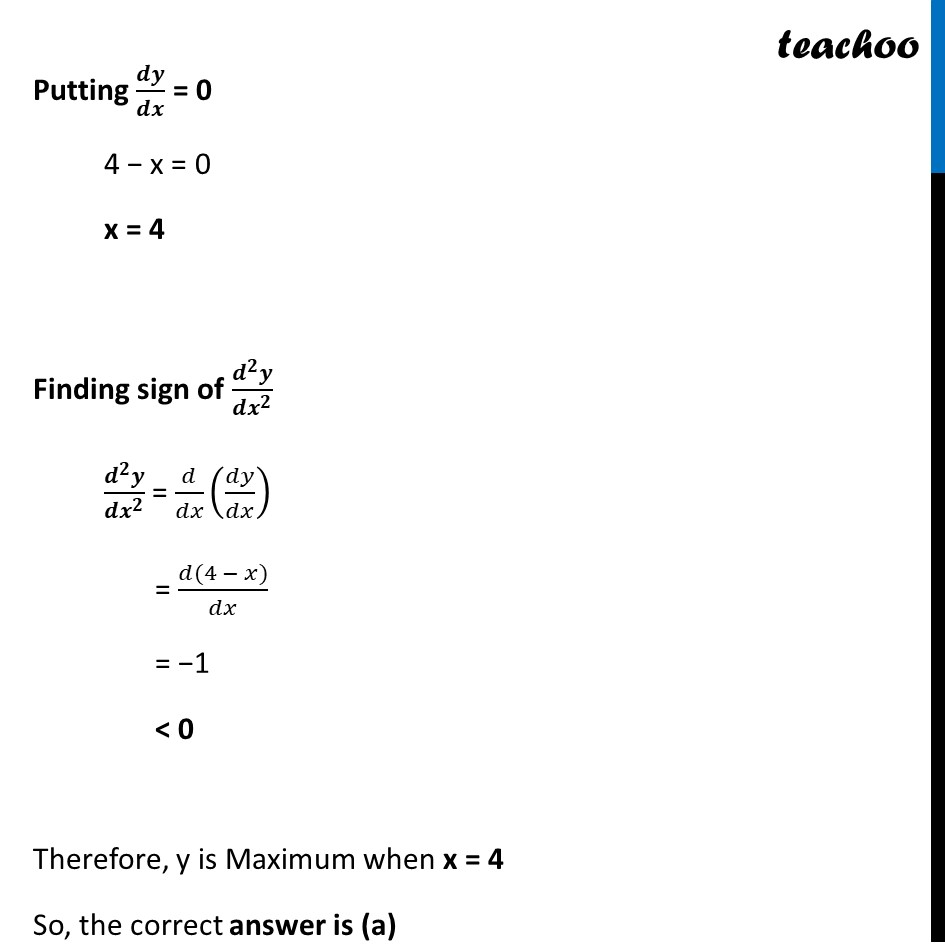## (d) 6 cm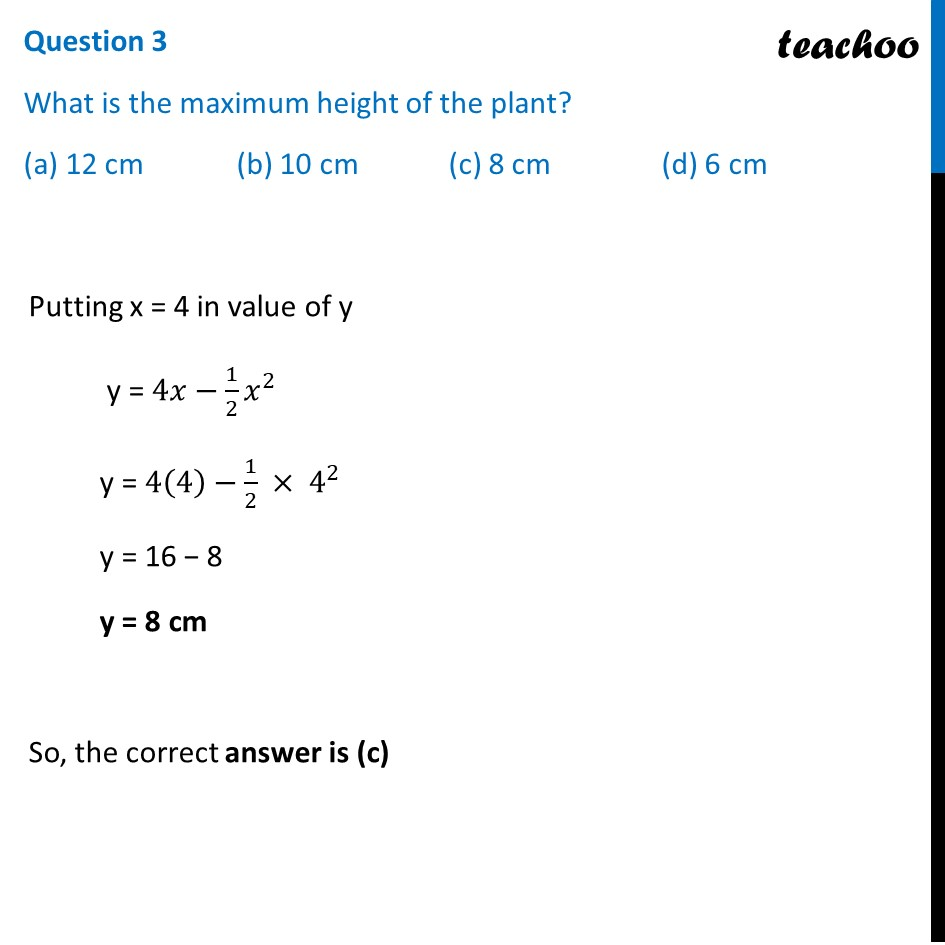## (d) 10 cm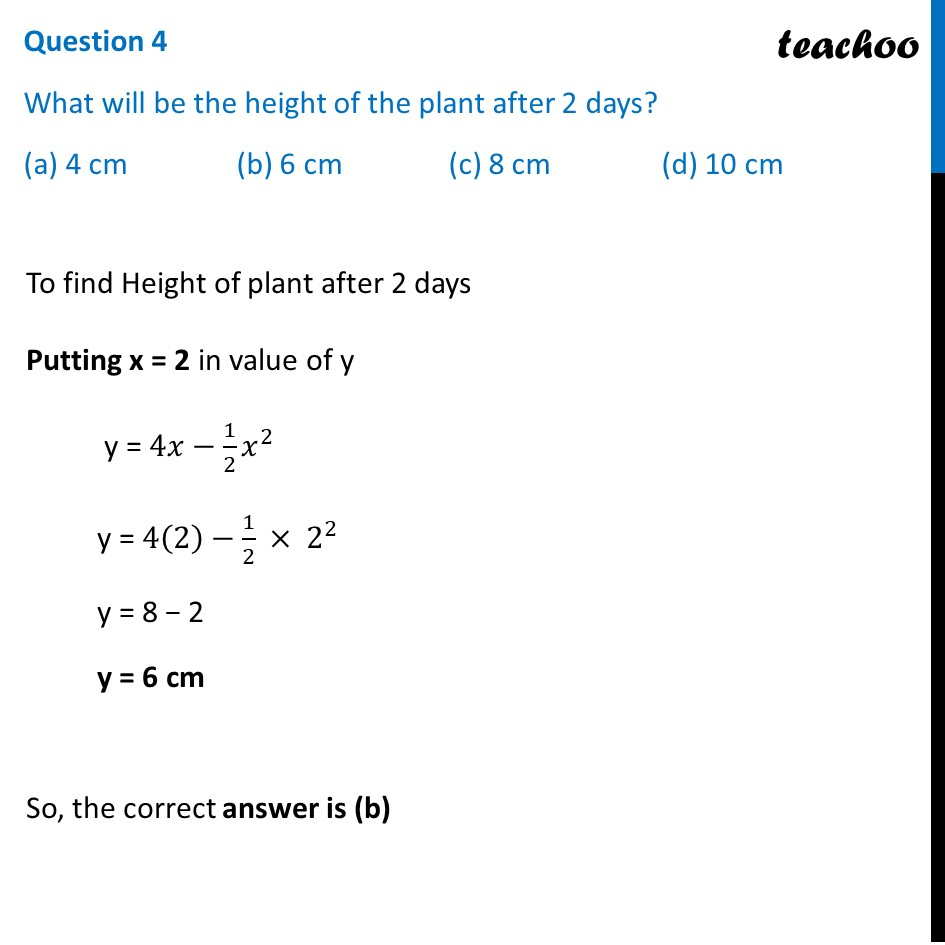## (d) 1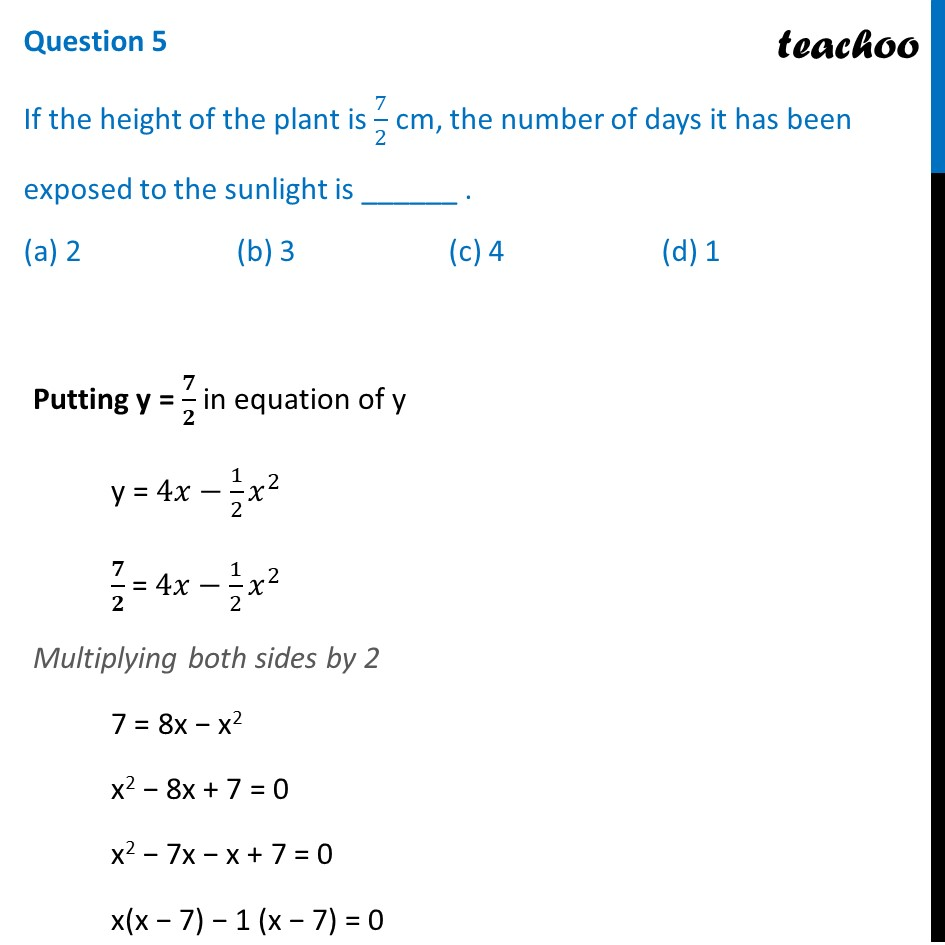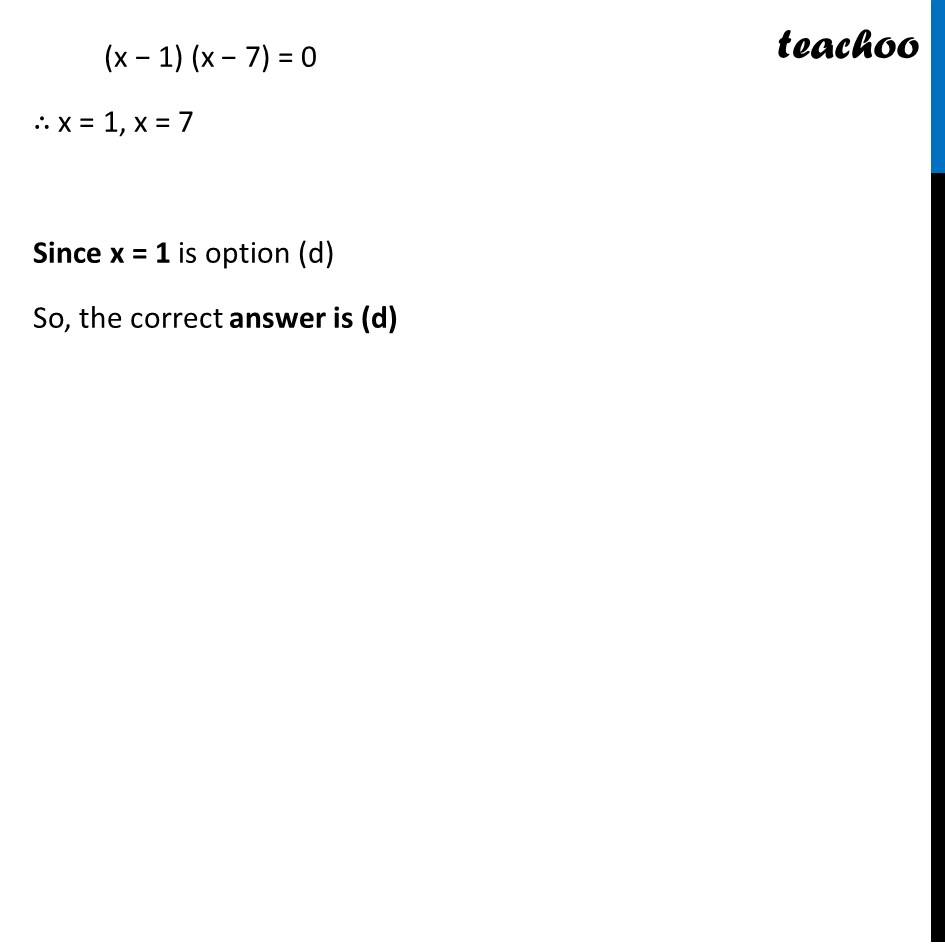Learn in your speed, with individual attention - Teachoo Maths 1-on-1 Class

### Transcript

Question The Relation between the height of the plant (y in cm) with respect to exposure to sunlight is governed by the following equation y = 4x – 1/2 𝑥^2 where x is the number of days exposed to sunlight. Question 1 The rate of growth of the plant with respect to sunlight is ______ . (a) 4𝑥 – 1/2 𝑥^2 (b) 4 – 𝑥 (c) 𝑥 – 4 (d) 𝑥 – 1/2 𝑥^2 Rate of growth of plant with respect to sunlight = 𝒅𝒚/𝒅𝒙 = d(4𝑥 − 1/2 𝑥^2 )/𝑑𝑥 = 4 − 1/2 × 2𝑥 = 4 – 𝒙 So, the correct answer is (b) Question 2 What is the number of days it will take for the plant to grow to the maximum height? (a) 4 (b) 6 (c) 7 (d) 10 Given y = 4𝑥−1/2 𝑥^2 We need to find value of x, when y is maximum Thus, we need to find Maximum value of y Finding Maximum value of y 𝑑𝑦/𝑑𝑥 = 4 − x Putting 𝒅𝒚/𝒅𝒙 = 0 4 − x = 0 x = 4 Finding sign of (𝒅^𝟐 𝒚)/(𝒅𝒙^𝟐 ) (𝒅^𝟐 𝒚)/(𝒅𝒙^𝟐 ) = 𝑑/𝑑𝑥 (𝑑𝑦/𝑑𝑥) = (𝑑(4 − 𝑥))/𝑑𝑥 = −1 < 0 Therefore, y is Maximum when x = 4 So, the correct answer is (a) Question 3 What is the maximum height of the plant? (a) 12 cm (b) 10 cm (c) 8 cm (d) 6 cm Putting x = 4 in value of y y = 4𝑥−1/2 𝑥^2 y = 4(4)−1/2 × 4^2 y = 16 − 8 y = 8 cm So, the correct answer is (c) Question 4 What will be the height of the plant after 2 days? (a) 4 cm (b) 6 cm (c) 8 cm (d) 10 cm To find Height of plant after 2 days Putting x = 2 in value of y y = 4𝑥−1/2 𝑥^2 y = 4(2)−1/2 × 2^2 y = 8 − 2 y = 6 cm So, the correct answer is (b) Question 5 If the height of the plant is 7/2 cm, the number of days it has been exposed to the sunlight is ______ . (a) 2 (b) 3 (c) 4 (d) 1 Putting y = 𝟕/𝟐 in equation of y y = 4𝑥−1/2 𝑥^2 𝟕/𝟐 = 4𝑥−1/2 𝑥^2 Multiplying both sides by 2 7 = 8x − x2 x2 − 8x + 7 = 0 x2 − 7x − x + 7 = 0 x(x − 7) − 1 (x − 7) = 0 (x − 1) (x − 7) = 0 ∴ x = 1, x = 7 Since x = 1 is option (d) So, the correct answer is (d)